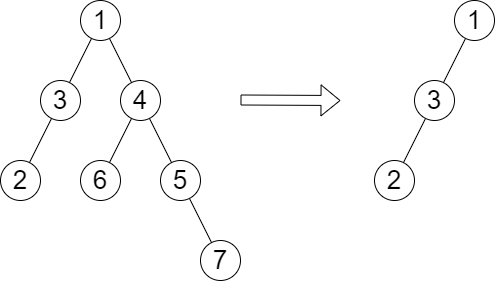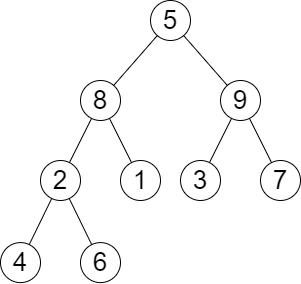2458. Height of Binary Tree After Subtree Removal Queries
Hard
483
13

You are given the `root` of a binary tree with `n` nodes. Each node is assigned a unique value from `1` to `n`. You are also given an array `queries` of size `m`.

You have to perform `m` independent queries on the tree where in the `ith` query you do the following:

• Remove the subtree rooted at the node with the value `queries[i]` from the tree. It is guaranteed that `queries[i]` will not be equal to the value of the root.

Return an array `answer` of size `m` where `answer[i]` is the height of the tree after performing the `ith` query.

Note:

• The queries are independent, so the tree returns to its initial state after each query.
• The height of a tree is the number of edges in the longest simple path from the root to some node in the tree.

Example 1:```Input: root = [1,3,4,2,null,6,5,null,null,null,null,null,7], queries = 
Output: 
Explanation: The diagram above shows the tree after removing the subtree rooted at node with value 4.
The height of the tree is 2 (The path 1 -> 3 -> 2).
```

Example 2:```Input: root = [5,8,9,2,1,3,7,4,6], queries = [3,2,4,8]
Output: [3,2,3,2]
Explanation: We have the following queries:
- Removing the subtree rooted at node with value 3. The height of the tree becomes 3 (The path 5 -> 8 -> 2 -> 4).
- Removing the subtree rooted at node with value 2. The height of the tree becomes 2 (The path 5 -> 8 -> 1).
- Removing the subtree rooted at node with value 4. The height of the tree becomes 3 (The path 5 -> 8 -> 2 -> 6).
- Removing the subtree rooted at node with value 8. The height of the tree becomes 2 (The path 5 -> 9 -> 3).
```

Constraints:

• The number of nodes in the tree is `n`.
• `2 <= n <= 105`
• `1 <= Node.val <= n`
• All the values in the tree are unique.
• `m == queries.length`
• `1 <= m <= min(n, 104)`
• `1 <= queries[i] <= n`
• `queries[i] != root.val`
Accepted
9.8K
Submissions
26.4K
Acceptance Rate
37.1%

Seen this question in a real interview before?
1/4
Yes
No

Discussion (0)

Related Topics